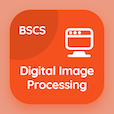Online CS Degree Courses

Digital Image Processing Quizzes

Digital Image Processing Quiz - Complete

Practice Point Line and Edge Detection in Image Processing quiz questions and answers, point line and edge detection in image processing MCQ questions PDF, test 111 to study Digital Image Processing online course. Image Segmentation MCQ questions, point line and edge detection in image processing Multiple Choice Questions (MCQ Quiz) for online college degrees. Point Line and Edge Detection in Image Processing Book PDF: erosion and dilation, introduction to wavelet and multiresolution processing, histogram processing, morphological analysis in image processing, point line and edge detection in image processing test prep for online computer engineering programs.

"Second derivatives in image segmentation produces" Quiz PDF: point line and edge detection in image processing App APK with thin edges, thick edges, fine edges, and rough edges choices for master's degree in computer science. Solve image segmentation questions and answers to improve problem solving skills for computer information science.

## Trivia Questions on Point Line & Edge Detection in Image Processing MCQs

MCQ: Second derivatives in image segmentation produces

thick edges
thin edges
fine edges
rough edges

MCQ: The translation of set B is the

{c|c = b+z}
{c|c = b-z}
{c|c = bxz}
{c|c = b}

MCQ: Digital image with intensity levels in the range [0,L-1] is called

k-map
histogram
truth table
graph

MCQ: Diagonally opposed filters is said to be

modulation
multiplier
cross modulation
subband coding

MCQ: In morphological operations SE is viewed as

low pass filter
high pass filter

### More Quizzes from Digital Image Processing Book